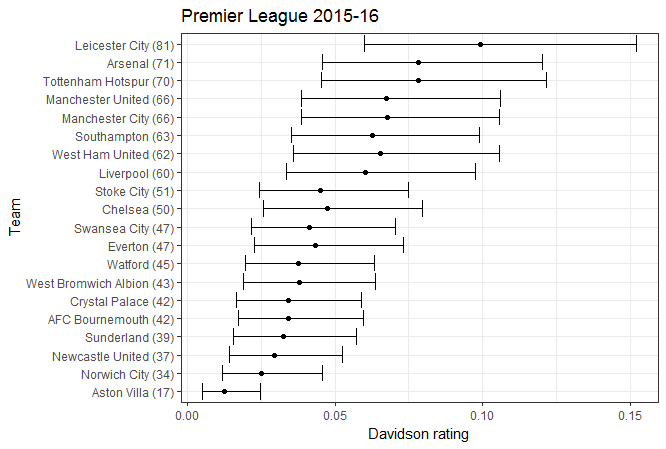# The Bayesian Bradley-Terry model with draws

In the previous post I tried out the Stan software to implement two Bayesian versions of the Bradley-Terry (BT) model. One drawback of the Bradley-Terry model is that it can’t handle draws, which seriously hampers its utility in modelling sports data. That was one reason I used handball results rather than football results as the example, since draws are rare in handball.

One (of several) extension of the BT model that can handle draws is the Davidson model. This was developed in the 1970 paper ‘On Extending the Bradley-Terry Model to Accommodate Ties in Paired Comparison Experiments‘. In short, the model adds a new parameter, $$\nu$$, which influences the probability of a draw. When $$\nu = 0$$, the model becomes the ordinary BT model.

In my Stan implementation below I use a Dirichlet prior on the ratings, like last time. The consequence of this is that the sum of all the ratings is 1. In the BT model this gives us the the nice interpretation that the rating the probability of a team of winning against an hypothetical average team. This property is not exactly carried over to the Davidson model, but a related property is. The ratio of two ratings, $$\pi_1 / \pi_2$$, is the probability that team 1 wins against team 2, applies to both the BT model and the Davidson model

In my implementation of the BT model I used the Bernoulli distribution to model the outcomes, which is appropriate when we only have two outcomes. As you can see from the code below, we now have to use the categorical distribution, since we now have three outcomes. I also use an exponential prior on $$\nu$$. Admittedly, I have no particular reason for this except that it is the traditional choice for parameters that has to have only positive values.

Anyway, here is the Stan code:

data {
int<lower=0> N; // N games
int<lower=0> P; // P teams

// Each team is referred to by an integer that acts as an index for the ratings vector.
int team1[N]; // Indicator arrays for team 1
int team2[N]; // Indicator arrays for team 1
int results[N]; // Results. 1 if home win, 2 if away won, 3 if a draw.

real<lower=0> nu_prior_rate;

vector[P] alpha; // Parameters for Dirichlet prior.
}

parameters {
// Vector of ratings for each player
// The simplex constrains the ratings to sum to 1
simplex[P] ratings;

// Parameter adjusting the probability of draw.
real<lower=0> nu;
}

model {

// Array of length 3 vectors for the three outcome probabilies for each game.
vector result_probabilities[N];
real nu_rating_prod;

ratings ~ dirichlet(alpha); // Dirichlet prior on the ratings.

nu ~ exponential(nu_prior_rate); // exponential prior on nu.

for (i in 1:N){

// nu multiplied by the harmonic mean of the ratings.
nu_rating_prod = sqrt(ratings[team1[i]] * ratings[team2[i]]) * nu;

result_probabilities[i] = nu_rating_prod / (ratings[team1[i]] + ratings[team2[i]] + nu_rating_prod);
result_probabilities[i] = ratings[team1[i]] / (ratings[team1[i]] + ratings[team2[i]] + nu_rating_prod);
result_probabilities[i] = 1 - (result_probabilities[i] + result_probabilities[i]);

results[i] ~  categorical(result_probabilities[i]);
}

}


Another thing I wanted to do this time was to do proper MCMC sampling, so we could get the Bayesian posterior credibility intervals. The sampling takes longer time than the optimization procedure I used last time, but it only took a few seconds to get a decent amount of samples.

For the reanalysis of the handball data from last time I set the Dirichlet prior parameters to 5 for all teams, and the rate parameter for the exponential prior on $$\nu$$ is 1. We can visualize the estimate of the ratings and their uncertainties (95% intervall) using a forest plot:The results agree with the ones from last time, but this time we also see that the credibility intervals are rather large. This is perhaps not that surprising, since the amount of data is rather limited. The posterior (mean) point estimate for $$\nu$$ is 0.15.

But let’s take a look at some English Premier League football data. With the ordinary BT model this would not work so well since there’s a lot of draws in football. Ignoring them would not be tenable. Below are the ratings, with 95% credibility interval, based on data from the 2015-15 season, using the same prior parameters as in the handball data set. The league points are shown in parenthesis for comparison.The ratings generally agree with the points, except in a few instances, where a team or two have switched places. Another interesting thing to notice is that the width of the intervals seem to be related to the magnitude of the rating. I am not exactly sure why that is, but I suspect its due to the fact that the ratings are in a sense binomial probabilities, and these are known to have greater variance the closer they are to 0.5.

The point estimate for $$\nu$$ is 0.85 for this data set. Compared to the 0.15 for the handball data, it is clear that this reflects the higher overall probability of draws in football. In the handball data set only 6 games ended in a draw, while in the football data set about 20% of the games was a draw.